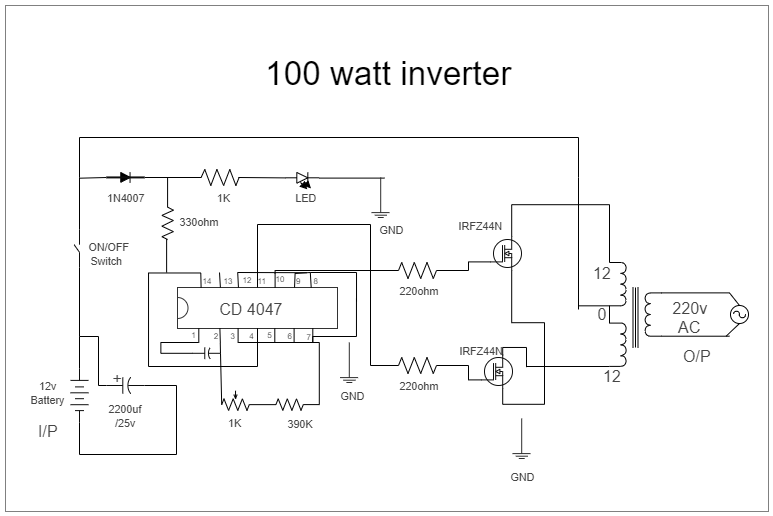Template Community / 100 Watt Inverter Circuit Diagram

# 100 Watt Inverter Circuit DiagramCharlotte
Published on 2021-03-02
Edit Online1. Introduction

Here is a circuit diagram example about the 100 Watt inverter. Circuit Diagram is a free application for making electronic circuit diagrams and exporting them as images. A circuit diagram is a graphical representation of a circuit. Illustrated circuit diagrams use simple images of components, while schematic diagrams use standardized symbolic representations to show the components and interconnections of the circuit. Check this circuit diagram, and make your own circuit diagram with ease.

2. What is Circuit Diagram

In simple terms, a circuit diagram is a visual representation of an electric connection. Symbols are usually used in a circuit diagram, making it easier for users to understand the connection. Of the different circuit diagrams, an ammeter circuit diagram is one of the easiest connections. Let's learn about it in detail here.

3. How to Create a Circuit Diagram

Creating a Circuit Diagram in EdrawMax Online is pretty simple. The free Electrical Circuit maker has several features as you can instantly import the images or icons from your system, Google Drive, or DropBox. Your Electrical Circuit diagram will require additional media content, making it more creative.

Step 2: Choose a template
EdrawMax comes with hundreds of free diagram templates. Select a pre-designed template by entering the Keyword in the ""Search"" section or exploring different diagram sets. In this case, you will find different types of Electrical Circuits diagrams under the ""Electrical Engineering"" section under the ""Engineering"" section. You will find different diagram sets, like Basic Electrical, Circuits and Logic, Industrial Control System, and Systems' Diagram. Alternatively, you can simply click on ""+"" in EdrawMax Online canvas to create a diagram of your preference.

Step 3: Work on your research
As per the symbols, a circuit diagram, also called an electrical diagram, elementary diagram, or electronic schematic, is defined as a simplified graphical representation of an electrical circuit. As you will see from the circuit diagram symbols, there are five main types of electrical circuits: Close circuit, open circuit, short circuit, series circuit, and parallel circuit. Each type of circuit is designed to create a conductive path of current or electricity.

Step 4: Customize the diagram
Customize your Circuit diagrams by changing the color or adding more relevant data. You can also add or remove the Electrical Circuit symbols or content accordingly based on your research. Since it is about electrical engineering research, you can add more relevant data about different circuit diagrams.

Step 5: Export & Share
Once your required circuit diagram is completed, you can share it amongst your colleagues or clients using the easy export and share option. You can further export the diagram in multiple formats, like Graphics, JPEG, PDF, or HTML. Also, you can share the designs on different social media platforms, like Facebook, Twitter, LinkedIn, or Line. In simple words, you can export your incredible Circuit diagrams to the files you want with just a few clicks.
Tag
circuit diagram
Report
2
1.8kPostRecommended Templates